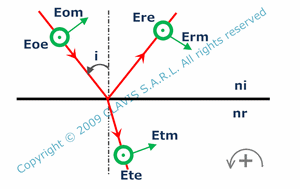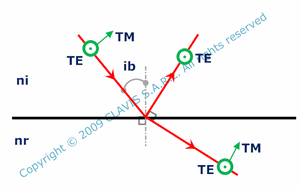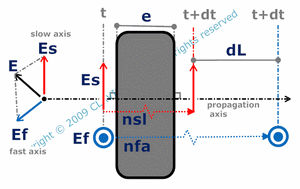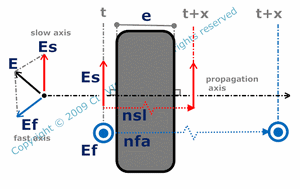and ortutorials

# polarization

Polarization basics

Light waves are electromagnetic waves modelized by two vectors propagating perpendicularly to their direction ( when considering a non birefringent propagation medium ). These vectors are perpendicular to each other. They represent respectively the electric field and the magnetic field. The polarization characterizes the electric or magnetic field direction. The polarization is linear when the direction of the electric field vector is invariant with time. The polarization is respectively circular or elliptical when the electric field vector describes respectively a circle or an ellipse with time. The polarization can also be randomatic. It's the case of unpolarized waves.

Polarization and dielectric interfacesThe reflection and transmission ratios at a dielectric interface depend on the polarization of the incident wave. For an incident wave with Eo as the electric field vector, Er and Et being the electric field vectors respectively of the reflected and transmitted waves , Eo, Er, Ep can be splitted respectively into Eoe, Ere, Ete their vector component parallel to the interface ( TE polarization ) and Eom, Erm, Etm their vector component in the incidence plane ( TM polarization ). re and rm are the reflection ratios by the interface respectively for TE and TM polarization. re = Ere/Eoe and rm = Erm/Eom. te and tm are the transmission ratios by the interface respectively for TE and TM polarization. te = Ete/Eoe and tm = Etm/Eom.

re, rm, te and tm are functions of the refraction index of the first medium( ni ), the refraction index of the second medium ( nr ) and the angle of incidence ( i ).Consequently, for a TE polarized wave, ratios of reflected and transmitted intensity Re and Te depend on ni, nr and i, as well as Rm and Tm, respectively reflected and transmitted intensity ratios for a TM polarized wave.

Brewster angleFor TM polarized light waves, there is a special angle of incidence called "Brewster angle" ( ib ) for which no light is reflected.

ib depends on the refraction index of the first medium ni and the refraction index of the second medium nr.

Birefringence

Birefringence is a property of certain materials. Non birefringent materials have an isotropic atomic structure. They transmit light waves in the same way whatever the polarization state. Birefringent materials have an anisotropic atomic structure. In the general case, a birefringent material splits an incident light wave in two waves with different polarization components that are refracted in two different directions ( double refraction ). Simplest birefringent materials ( uniaxis ) have a privilegiate axis called ordinary axis. For light waves polarized in a plane perpendicular to this axis ( ordinary wave ), the refraction index has a certain value no ( ordinary index ). For light waves linearly polarized parallel to the ordinary axis ( extraordinary wave), the refraction index has a different value ne (extraordinary index).Considering a birefringent plane parallel plate, in the particular case where ordinary axis is parallel to its interfaces and for normal incidence, double refraction occurs but no deviation is observed. However, difference of optical paths between extraordinary and ordinary waves dL can be calculated as a function of no, ne and plate thickness e. Generally, fast and slow axis with respective index nfa and nsl are used instead of ordinary and extraordinary axis.

nfa = ne and nsl = no when ne is smaller than no.
nfa = no and nsl = ne when ne is larger than no.Indeed, phase shift between slow and fast axis can be calculated for a monochromatic wave as a function of nfa, nsl, e and the wavelength lam.

References

"Optique Fondements et applications" - 2004 - author : José-Philippe Perez.

"Cours d'optique physique" - Institut d'optique théorique et appliquée - 1985 - author : Christian Imbert.

"Cours d'optique ondulatoire" - Université Denis Diderot Paris 7 - 2006 - author : G.Rebmann.

"Études graphiques des propriétés optiques des lames minces" - Journal de physique - 1950 - author : D. Malé.